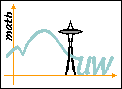# Research Training Group Inverse Problems and Partial Differential Equations

## RTG Principal Investigator/Director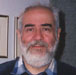Gunther Uhlmann has been working on an array of inverse problems since the 1980s. Some are related to partial differential equations, like the EIT problem; others arise from differential geometry, like the boundary rigidity problem. His theoretical work on cloaking also makes connection to material science. In 2011 the AMS awarded Gunther the Bôcher Prize.

## RTG co-Principal Investigators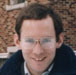Robin Graham is a geometric analyst, one of whose main interests is conformal geometry. He has worked on scattering theory and Dirichlet-to-Neumann maps in the setting of asymptotically hyperbolic manifolds, with connections to the AdS/CFT correspondence in string theory. Among other topics, he is presently studying the inverse problem of boundary rigidity for asymptotically hyperbolic metrics, in collaboration with Uhlmann and P. Stefanov.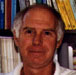Jim Morrow has been the director since 1988 of the REU program at UW in Discrete Inverse Problems. Since 1994 he has been director of Mathday at UW, a program which brings over 1,200 high school students to campus for a day of mathematical discovery. He also is co-director of SIMUW (Summer Institute for Mathematics at the University of Washington).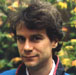Hart Smith works on the application of harmonic analysis to partial differential equations. The current focus of his research involves using wave packet methods to study the behavior of travelling waves and eigenfunctions on compact manifolds. He pioneered the use of curvelets to study wave propagation in rough media. In recent collaboration with Uhlmann and de Hoop, these curvelet techniques were applied to the development of computational schemes for the seismic imaging problem.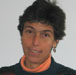Tatiana Toro works at the interface of partial differential equations and geometric measure theory. Her recent work concerns free boundary regularity problems with rough data, and has settled several questions concerning the relationship between the geometry of a set and the regularity of the measures it supports. Tatiana spoke at ICM 2010 in Hyderabad, India.

## RTG Postdoctoral Fellows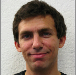Jonas Azzam works in the fields of geometric measure theory and analysis on metric spaces. His research uses multi-scale analysis and techniques and methodology from harmonic analysis to quantitatively study the geometry of subsets of Euclidean space and general metric spaces. Recently, his work has focused on quantitative rectifiability and Lipschitz analysis.Brent Werness studies the Schramm-Loewner Evolutions, a topic which incorporates ideas from probability theory and complex analysis to study the geometry of curves in models from two-dimensional statistical physics. Recently, he has approached these problems using techniques from rough path theory, and quasi-conformal geometry.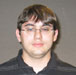Matthew Badger completed his Ph.D. under Tatiana Toro. While supported by the RTG grant he studied geometric measure theory and its application to PDE, specifically harmonic measure on non-tangentially accessible domains in higher dimensions. A focus of his research was to prove analogues in space of results in the plane by using geometric measure theory to replace complex analysis. He now has a position at Stony Brook University.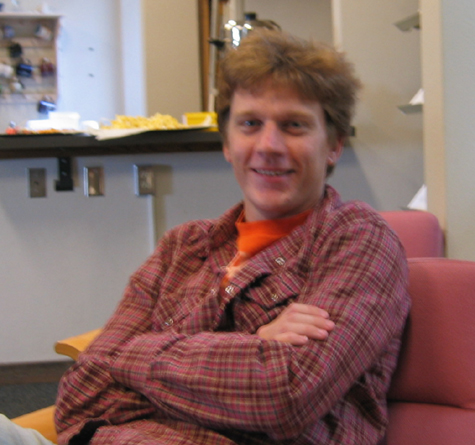Joel Barnes is a third-year graduate student studying probability theory with Krzysztof Burdzy. His current research concerns obtaining solutions to a free-boundary parabolic PDE as the hydrodynamic limit of an interacting particle system. The system is connected to an alternate model of exclusion dynamics with a different stationary distribution than the traditional simple exclusion process.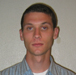Gregory Drugan is a fourth year graduate student in the math department. He is interested in differential geometry and partial differential equationsand is currently working on problems involving geometric flows. His thesis advisor is Yu Yuan.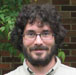Sean Holman works in the area of inverse problems. The general objective in such problems is to determine parameters of a medium based on measurements made around the boundary. In his graduate work he considered the problem of recovering anisotropic media based on polarization measurements of light rays passing through them. In winter 2010 he began a position at Purdue University, working on geophysical inverse problems.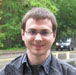Mark Hubenthal is interested in numerous topics related to inverse problems including different imaging methods, invisibility, inverse transport, and scattering. He is currently working on photo-acoustic tomography, which is closely related to the inverse Schrodinger problem with internal measurements. One particular question of interest concerns uniqueness and stability while restricted to using only illuminations supported on part of the boundary of the medium. Mark's Ph.D. advisor is Gunther Uhlmann.Stephen Lewis is a third year graduate student studying geometric measure theory and PDE with Tatiana Toro.Stephen McKeown is a second year Ph.D. student. He is interested in pure differential geometry and its interactions with theoretical physics.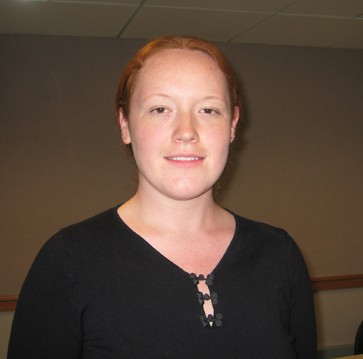Lee Patrolia is a PhD student working on inverse problems and PDE. She is currently working on aspects of photo- and thermo- acoustic tomography and inverse problems for the linear transport equation. In particular, she is investigating an inverse problem for the stationary linear transport equation on a Riemannian manifold. Her thesis advisor is Gunther Uhlmann.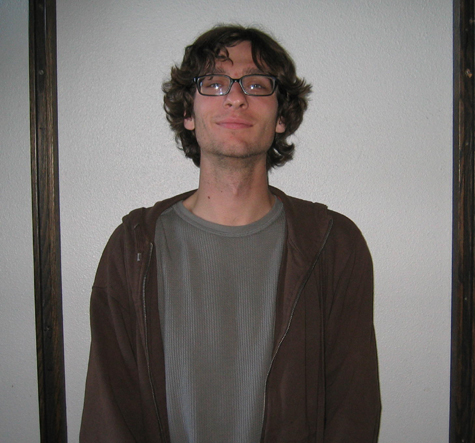Justin Tittelfitz is currently in his fourth year of the graduate program. He is primarily interested in inverse problems involving the elastic wave equation, especially those with applications to medical and seismic imaging. One particular goal is the recovery of an elastic wave's initial displacement via boundary measurements. Justin's Ph.D advisor is Gunther Uhlmann.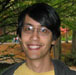Joshua Tokle works on the analytic side of probability theory. Under his advisor, Zhen-Qing Chen, he is working to establish two-sided estimates for the heat kernels of symmetric alpha-stable processes and censored stable processes in domains with some boundary regularity conditions.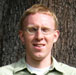James Vargo works on inverse problems related to boundary rigidity. In these problems the aim is to reconstruct a Riemannian metric in the interior of a manifold from measurements of the geodesics taken at the boundary. This problem is an abstract model for travel time tomography in which one tries to reconstruct wave speeds inside a medium from travel times between boundary points. Jim now has a position at Texas A&M University.Ken Bube's research is at the interface between numerical analysis, partial differential equations, and geophysical imaging. In ongoing projects with geophysical researchers in industry, he is working on numerical algorithms for ill-posed problems, including seismic traveltime tomography and multiple removal. His work involves theoretical analysis of problems with non-unique solutions, coupled with numerical algorithms to regularize these problems.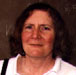Anne Greenbaum's research involves numerical linear algebra and the numerical solution of partial differential equations. Particular interests include nonnormal matrices, iterative methods for linear systems, and integral equations.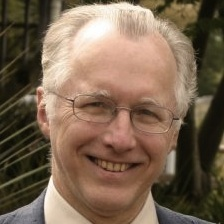Jack Lee is a geometric analyst whose research in recent years has centered on Riemannian geometry and general relativity. Lee is also the author of several widely used graduate texts in differential geometry.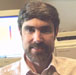Randall LeVeque developes and uses high-resolution finite volume methods to solve hyperbolic partial differential equations arising in fluid dynamics and wave propagation problems, including further development of the CLAWPACK software (www.clawpack.org). There are applications in geology --- tsunamis, volcanoes, landslides --- and in biomedicine: shock wave therapy and lithotripsy.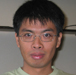Tu Nguyen's current interest is inverse problems, in particular the partial data inverse problem. He has worked on unique continuation, local well-posedness of dispersive equations, and Lagrangian mean curvature flows.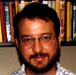Daniel Pollack's research centers on the applications of partial differential equations and analysis to problems in geometry and physics. In recent years his work has concerned solutions of the Einstein field equations of mathematical general relativity. For an introduction to these topics, and a list of open problems, see his co-written Mathematical general relativity: a sampler.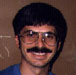John Sylvester researches the development of multi-dimensional mathematical tools for remote sensing applications. A primary emphasis is to draw precise and useful conclusions from data that is both limited and noisy. The mathematical problems underlying these remote sensing problems are the Inverse Scattering and Inverse Source problems.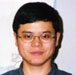Yu Yuan works in partial differential equations and differential geometry. His recent research focuses on fully nonlinear equations such as special Lagrangian equations (which include Monge-Ampere equations).